# Low-Stress and Efficient Design of Integrated Boost Series Parallel Fly-Back Converters

Low-Stress and Efficient Design of Integrated Boost Series Parallel Fly-Back Converters
Department of EEE, JNTUA College of Engineering, Ananthapuram, AP-515001, India

Department of EEE, G. Pulla Reddy Engineering College, Kurnool, AP-518346, India

Corresponding Author Email:
sravan_257@gnits.ac.in
Page:
393-401
|
DOI:
https://doi.org/10.18280/jesa.530310
7 April 2020
|
Accepted:
20 May 2020
|
Published:
31 July 2020
| Citation

OPEN ACCESS

Abstract:

This paper Presents comparative analysis of IBSPFC &QSC Based IBSPFC by using FPGA. By using this IBSPFC the voltage stress is reduced across the switch when compare to conventional fly-back converters. The multi winding transformer gives higher reliability i.e. if any one of the winding damages other one supplies power to the load. On the other hand, QSC based IBSPFC will achieved Zero voltage & Zero current switching, which will increase more efficiency when compare to conventional single stage integrated converters and IBSPFC. Operating modes of QSC, based IBSPFC&IBSPFC have been introduced. The primary side winding of the fly-back transformer is coupled in series across with bulk capacitor to minimize switch voltage stress and the secondary winding of the 1:1 fly-back transformer. Basic voltage mode control Techniques are used to control the output voltage and current. An input voltage of 25V primary side and 50V battery at secondary side, 100v output, 100W and 100KHz DC-Dc isolated QSC based IBSPFC is implemented using FPGA SPARTAN6LX9 and experimental results and comparative table have been presented.

Keywords:

Integrated Boost Series Parallel Fly-Back Converter (IBSPFC), QSC (Quasi Switched Capacitor), voltage mode control

1. Introduction

Existent Solar Power creation has addition leads in finding and running price different to more energy sources. Solar power is a clean method with nil least preservation. Mainly PV cells can be built on the topmost of apartments or homes and trade in metropolitan cities. In solar power, there is chance of irradiation fluctuation, which makes use of battery storage system to provide the required power to load . Due to separate sources, an individual power electronic converter is required which increase the complexity of the circuit shown in Figure 1 (a). To reduce the cost and complexity of the circuit a multi input dc-dc converter is preferred  which are shown in Figure 1(b). Presently dual input topologies are classified into two ways. One is non-isolated another one is isolated [2-14]. The basic non-isolated topologies of dc-dc converters are again classified as Buck, Boost and Buck-boost converters suffering from isolation and at the load side it requires large AC Transformers [3, 4]. Otherwise, the utility grid may affect leakage current in between solar panels and earth. The isolated converters of dual input dc/dc help to avoiding the line frequency transformer [5, 6]. The basic isolated dc/dc converters are fly back, half bridge, forward, push pull and full bridge converters [7-9]. To transfer the power from multiple sources to the output a separate isolated transformer is required for each source and the number of switches are increased followed by cost [10-15]. To eliminate this problem a two winding transformer is used [16-24]. By losing the isolation between the energy sources of single side of the isolation transformer the total number of switches in this type of topologies are reduced and Battery is connected at secondary side of the isolation transformer, the battery does not require any isolation to the output of the load [25-32].

Therefore, this type of topology is better than using multiple transformers. As the application in this paper is 100w or 200w, hence fly back converter is chosen. The basic fly back converter requires two snubber circuits one is at primary winding of transformer another one is at switch, which is connected in series with the transformer.

Generally, the fly back converter duty cycle is limited to 0.5.

To eliminate these problems IBSPFC and QSC based IBSPFC is preferred for 100w or 200w applications. In this paper single switch IBSPFC and QSC based IBSPFC is introduced. The main advantage of QSC based IBSPFC is it will reduce voltage stress on the switches, high reliability and ZVS and ZCS will achieve hence efficiency of the converter is improved .

The research study along with experimental results of IBSPFC with QSC is presented in this paper for closed loop control. The design considerations for inductor in boost circuit, quasi switched capacitor and output capacitor are evaluated. This presents design of QSC based IBSPFC operating at 100 kHz with DC input voltage magnitude of 25V on primary side, producing 50V and 100V at secondary side and at load with 100W of output power. The operating modes of IBSPFC are presented in Section 2 Present Necessity of IBSPFC and QSC based IBSPFC. Section 3. Section 4 presents mathematical Analysis; experimental results of the proposed circuit are shown in Section 5 for closed loop, Section 6 Working Methods of QSC Based IBSPFC. Section 7 Mathematical Calculations of QSC based IBSPFC. Section 8 Experimental Results of QSC based IBSPFC. Section 9 conclusion. and References related to the performance of the proposed circuit is discussed in this above Sections.

## 1.png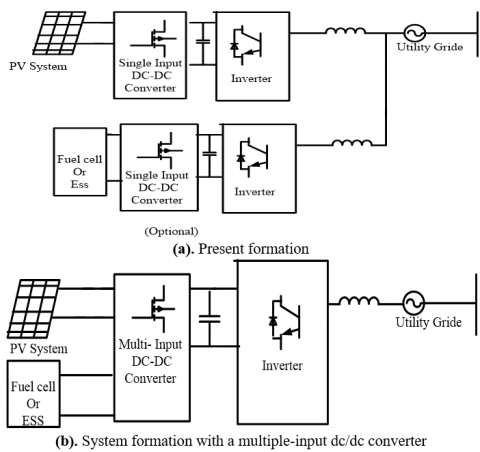Figure 1. Different system configurations with both PV and Fuel cell or ESS

2. Necessity of IBSPFC and QSC Based IBSPFC

## 2.png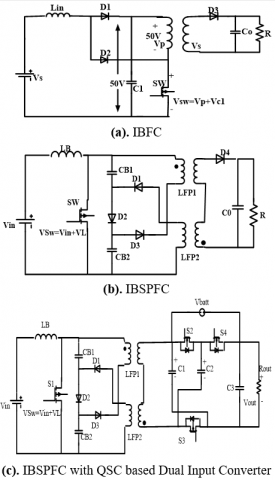Figure 2. Different topologies Boost Fly-back converter

Figure 2 Shows the different topologies of Boost Flyback converter and the Figures shown below 2(a) is Integrated Boost fly back converter (IBFC) but here the problem is the voltage across the switch is Vsw=Vp+Vc1. Let us consider one example that Vs=25V, D=0.5V&Vc1=50V (boost operation) and Vp=50V then the switch voltage becomes Vsw=Vp+Vc1=100v and in transient period the voltage may goes to 10kv hence switch may go to damage. To overcome this problem IBSPFC is used.

In this IBSPFC shown in Figure 2(b) the voltage stress is reduced because Vsw=VIN+VLB Let us consider one example VIN=25V, D=0.5&Vsw=VIN+VLB=50V, only and the capacitors CB1 and CB2 will acts as snubber circuit as well as bulk capacitor energy storage. The reliability is also present in this circuit because if any one of these capacitors or if any one of the fly-back transformers will damage the other transformer will transfers the half of the power to the load. To improve the efficiency and power transfer QSC based dual input configuration is used shown in Figure 2(c). The main advantage is Soft switching is implemented for both primary and secondary MOSFETS.

3. Operation of IBSPFC

The main circuit diagram of IBSPFC shows in Figure 3. The functioning modes in single switching cycle can be indicated in four intervals, shown in Figure 4 and the pulse waveforms and the corresponding current waveforms are shown in Figure 5.

In mode 1 [0 < t < t1: Figure 4(a)]: When the SW is in on state then the input current start flowing through +Vin – LB – SW – (-Vin). Therefore, inductor current starts increasing and no current flows through the remaining part of the circuit.

In mode 2 [t1< t < t2: Figure 4 (b)] when the switch is in off state, then the current flows through +VIN - LB - CB1 - D2 - CB2 - (-VIN) and the inductor LB discharges and capacitors CB1 and CB2 will charge through diode D2.

In mode 3 [t2< t < t3: Figure 4(c)] the switch is again turned on and the supply current flows through +Vin- LB - SW – (-Vin). And the small amount of current flows through +CB1 - SW – Lfp2 – D1 – (-CB1) and +CB2 – D3 – Lpf1 - SW – (-CB2). Due to reverse polarity of dot convention of fly-back transformer diode D4gets forward biased and the current flows through the output capacitor C0 and load resistance R.

In Mode 4 [t3< t < t4: Figure 4(d)] the switch is again turned off the bulk capacitors CB1 and CB2 get charged through +VIN - LB - CB1 - D2 - CB2 - (-VIN) and +Lpf1- CB1- D2 – D3 – (-Lpf1) and +Lfp2 – D1 – D2- CB2 – (-Lfp2). Due to reverse polarity of dot convention diode D4 gets reverse biased then the output capacitor C0 will discharges its energy to the load R.

## 3.png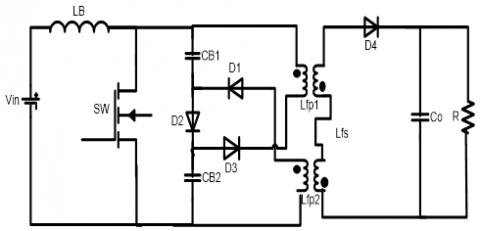Figure 3. Integrated Boost Series Parallel Fly-back converter (IBSPFC)

## 4.png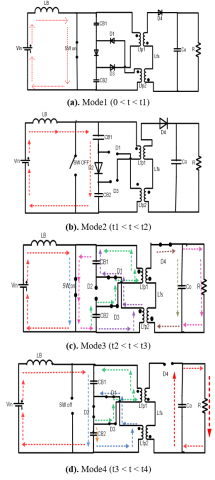Figure 4. Functioning modes of IBSPFC

## 5.png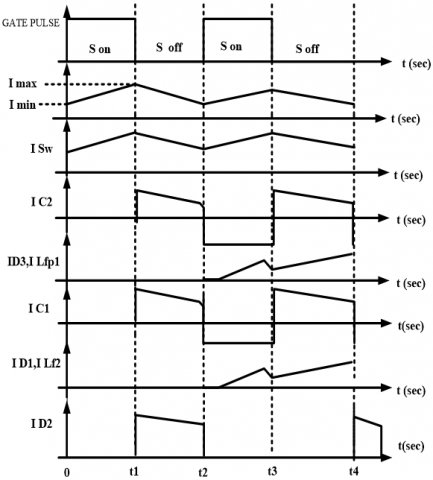Figure 5. Current waveforms

4. Mathematical Analysis

Input voltage VS = 24 V

Output Voltage V0 = 48 V

Output Current I0 = 1.2 A

Output Power P0 = 48 × 1.2 = 58 W

R= 40 Ω

For the step changer R = 65 Ω

$V_{0}=\frac{V_{S}}{(1-D)}\therefore D=0.5$                (1)

\begin{aligned} I_{S} &=\frac{I_{0}}{(1-D)} =2.4 \end{aligned}         (2)

But practically

IS=3.4A is Obtained

$\mathrm{n}=\frac{O U T P U T}{I N P U T}=\frac{58}{(24 * 3,4)}=68.2 \%$          (3)

To improve the efficiency & More Reliability QSC Based IBSPFC is used.

5. Experimental Results

Experimental results are shows Figure 6(a). Home based kit, Figure 6(b): Output voltage and current (48 v n 1.2 amp), Figure 6(c): Capacitor Voltage (24 volts), Figure 6(d): Primary voltage and Current (24 volts n 2 amp), Figure 6(e): Secondary Voltage (48v only due to symmetrical), Figure 6(f): Gate pulse and inductor current (3.15Amp) and Figure 6(g): Switch voltage.

## 6a.png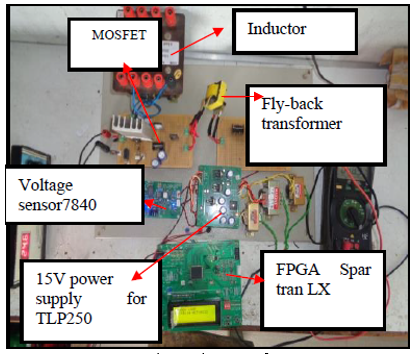(a). Experimental set up of IBSPFC

## 6b.png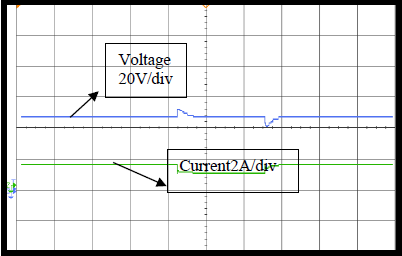(b). Output voltage and current (48 V n 1.2 A)

## 6c.png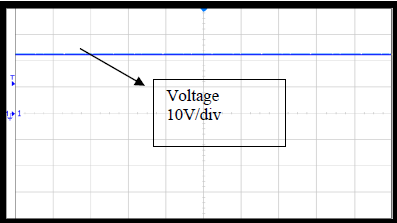(c). Capacitor Voltage (24 V)

## 6d.png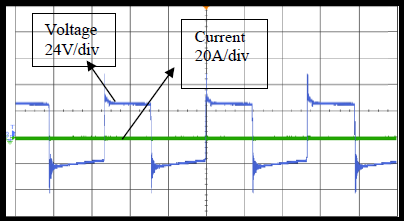(d). Primary voltage and Current (24 V n 2 A)

## 6e.png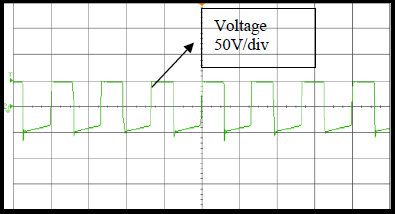(e). Secondary Voltage (48V only due to symmetrical)

## 6f.png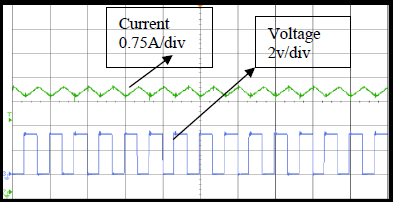(f). Gate pulse and inductor current (3.15A)

## 6g.png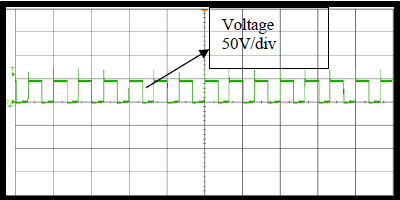(g). Switch voltage

Figure 6. Output DC-DC closed loop waveforms

6. Working Methods of QSC Based IBSPFC

Figure 7 shows the circuit diagram of QSC based IBSPFC. The working methods in particular switching cycle can be indicated in two-time intervals, shown in Figure 8 (a) & 8(b) and the corresponding waveforms are shown in Figure 9(a) &9(b).

In mode 1(M1) [0< t < t1: Figure 8(a)]: When the switches S1, S2, S3 are in ON condition. The current starts flowing through primary (Vin-L-S1) Therefore the boost inductor current starts increasing. Capacitor C1’C2’ will be discharging through (C1’-S1-D1) & (C2’-D3-S1) and supplied to load . On secondary side C1, C3 will be charging and C2 will be discharging though (C2-S2-Vbatt-C3-S3-C2).

In mode 2(M2) [t1< t < t2: Figure 8(b)] the switch S1, S2, S3 are switched off and S4 switched on. In this mode of operation, the current flows through the path of +Vin –L-C1’-D2-C2’-(-Vin). In this mode, the discharging of boost inductor takes place through the capacitors and diodeD2.and on secondary side capacitor C1, C3 are discharging and C2 is charging through-C2-Vbatt-S4-(+C2).

## 7.png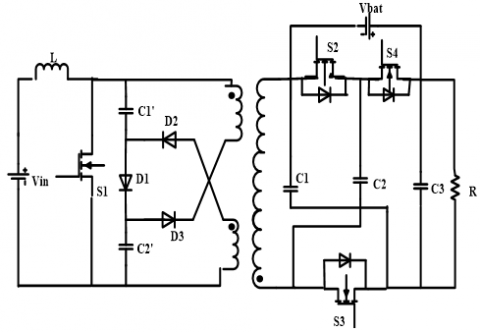Figure 7. QSC based IBSPFC

## 8.png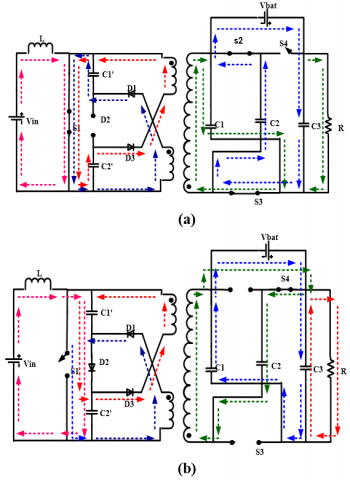Figure 8. (a) Mode1 (0 < t < t1); (b) Mode 2 (t1 < t < t2)

## 9.png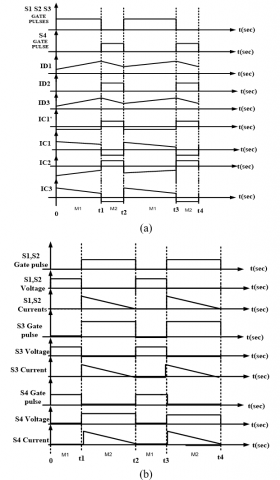Figure 9. (a) Waveforms -1; (b) waveforms - 2

7. Mathematical Calculations QSC Based IBSPFC

Mathematical calculations are obtained for the QSC based IBSPFC. Design specifications are Vin=25V, VO=100V, IO=1A, R=100Ω, fS=100 KHz, PO=100W, VB=50V and Battery=50V.The design calculations for QSC based IBSPFC are presented below based on the assumption that primary side source and secondary side battery will share total load.

The Boost Convertor Output Voltage (VB), Input Current (IL), Boost Inductance (LB), Fly-back inductance (LF), Bulk Capacitors CB1, CB2 and output filter capacitor (Co) are given as follows [34-37].

Boost Output Voltage:

$V_{B}=\frac{V_{i n}}{(1-D)} \therefore D=0.5$           (4)

Boost Inductor current:

\begin{aligned} I_{S} &=\frac{I_{O b}}{(1-D)} =1 A \end{aligned}        (5)

where, Iob is the Boost Converter Current delivery and Practically Is =3A is obtained for 100W. From the Boost Converter design Assume $\Delta i=0.05$.

Boost Inductance:

$L_{B}=\frac{V_{B} D}{f_{S} \Delta i}$            (6)

$L_{B}=3.5 \mathrm{mh}$              (7)

Bulk Capacitance:

$C_{B}=\frac{D}{\left(2 * f_{\mathrm{s}} * R\right)}$        (8)

$C_{B}=25 n f$         (9)

An approximation value of 100μF is chosen for practical case.

From the Fly-back Converter:

$P=\frac{V_{B}^{2} D^{2}}{\left(2 * L_{F} * f_{S}\right)}$           (10)

$L_{F}=53 \mu H$         (11)

An approximation value of 40 to 50μH is chosen for practical case and the Secondary side Capacitors Values.

$C_{1}=C_{2}=C_{3}=100 \mu F$          (12)

8. Experimental Results of QSC Based IBSPFC

Figure 10 shows the experimental setup of QSC Based IBSPFC.

## 10.png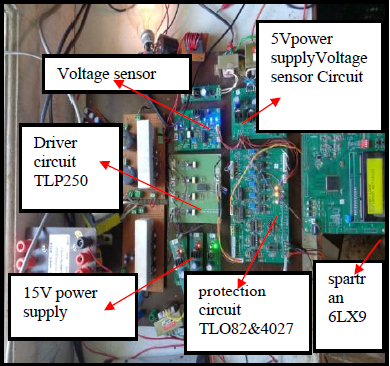Figure 10. Experimental setup of IBSPFC with QSC

Figure 11 shows Zero voltage, Zero current Switching and Gate pulse of S2. Here the duty cycle is 50% and it is obtained from the FPGA SPARTRAN 6. From the FPGA the pulse magnitude is only 5V. But for the MOSFET minimum voltage requirement is 15V and to achieve this TLP250 driver circuit is used. It can be seen that the voltage across the switch S2 will reaches zero once the gate pulse reaches the threshold voltage value. Also, the switch current magnitude is zero. Again, when the gate pulse will reach zero the voltage across the switch will increases and the current becomes almost zero. Therefore, Switch S2 realises ZVS ON, ZVS OFF, ZCS ON and ZCS OFF. The same will be applicable for switches S3 and S4 also. Figure 12 shows Zero Voltage, Zero Current Switching S3 and Gate pulse of S3, Figure 13 shows Zero voltage, Zero Current switching and Gate pulse of S4, Figure 14 shows Output Current 2A when the load is connected, Figure 15 shows Output Voltage 100V for a small change in load the voltage variation is also shown in Figure 15, Figure 16 shows Input Source Current 3A when the load is connected, Figure 17 shows Battery input Current 0.7A. Figure 18 represents efficiency curves of IBSPFC and QSC based IBSPFC. Table 1 shows the hardware details of DC-DC Converters.

## 11.png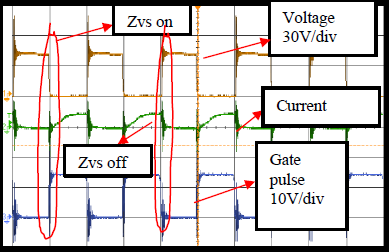Figure 11. Zero voltage, Zero Current Switching S2

## 12.png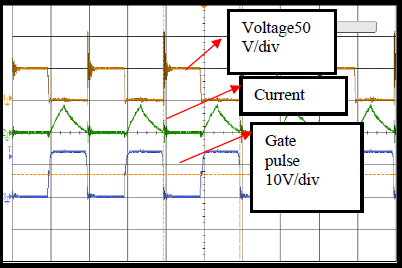Figure 12. Zero Voltage, Zero Current Switching S3

## 13.png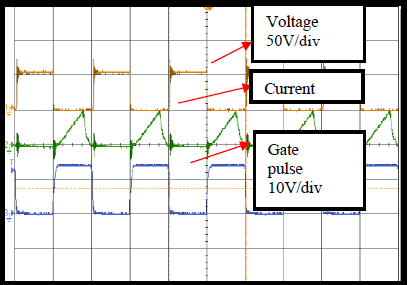Figure 13. Zero voltage, Zero Current switching S4

## 14.png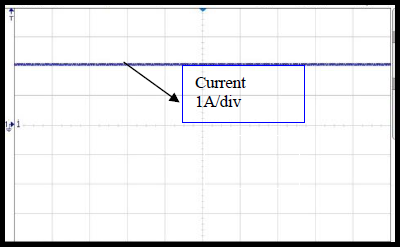Figure 14. Output Current 2A

## 15.png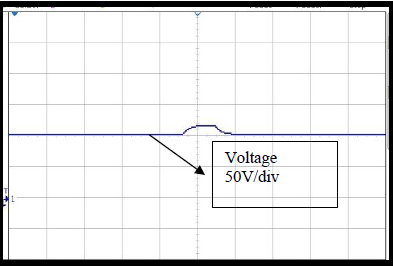Figure 15. Output Voltage 100V

## 16.png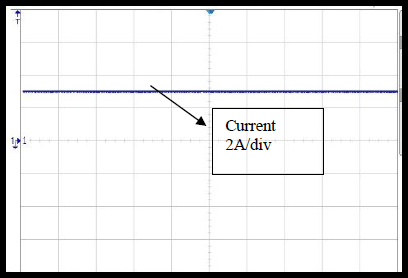Figure 16. Input Source Current 3A

## 17.png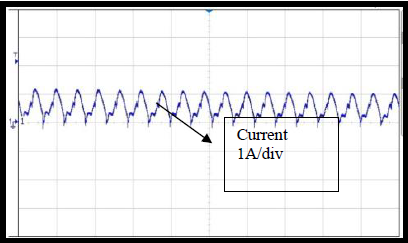Figure 17. Battery input Current 0.7A

## 18.png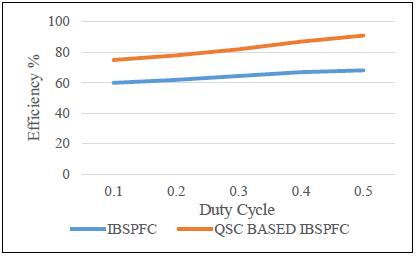Figure 18. Efficiency curves of IBSPFC and QSC Based IBSPFC

Table 1. Hardware details

 Name Number Ratings Inductor 100Khz,1m 10A MOSFET K2611 Toshiba 900V, 9A Diode MUR3060 600V,30A Capacitors (Primary) 63V,100μF Capacitors (Secondary) 100μF,100V Transformer (ratio) 1:1 50V/50V Transformer Inductance 45μH Protection circuit TL082 Spartan 8LX

Table 2. Comparison of IBSPFC & QSC Based IBSPFC
 IBSPFC QSC Based IBSPFC Less Reliability when compare to QSC Based IBSPFC High Reliability when compare to IBSPFC Up to100W Up to 200W Efficiency is 68.2% Efficiency is 91% No Snubber Circuit is Required No Snubber Circuit is Required

The Table 2 shows comparision of IBSPFC and Qsc Based IBSPFC. Here the IBSPFC reliablity is high when compare to conventional flyback converter, because of using mid-point transformer though if any one of the winding damages the power can be transferred with other winding of the transformer and also les swithing stress. But when compare to QSC Based IBSPFC the reliability is less because QSC based IBSPFC used to power supplies therefore if any power supply is not working other one will supply the power to the load.
9. Conclusion

This paper presents analysis and working methods of IBSPFC and QSC based IBSPFC. With this proposed connection of boost converter and fly back converter, the observations made are low switch voltage stress, tight voltage regulation with which the efficiency is improved. For the input voltage levels of 25 V, 50 V an output voltage of 100 V is obtained with the help of developed proto type which produces an output power of 100 w at switching frequency of 100 KHz. The converter has the ability to operate at 200 w also. The applications of this developed model are battery charging and series connection LED drives etc. With the combination of IBSPFC with QSC, the efficiency of the convertor increases that i.e. 91% and because of soft switching used in QSC helps to increase overall converter efficiency.

References

 Omran, W.A., Kazerani, M., Salama, M.M.A. (2011). Investigation of methods for reduction of power fluctuations generated from large grid-connected photovoltaic systems. IEEE Trans. Energy Conversion, 26(1): 318-327. https://doi.org/10.1109/TEC.2010.2062515

 Solero, L., Lidozzi, A., Pomilio, J.A. (2005). Design of multiple-input power converter for hybrid vehicles. IEEE Trans. Power Electron, 20(5): 1007-1016. https://doi.org/10.1109/TPEL.2005.854020

 Khaliah, A., Cao, J., Lee, Y. (2009). A multiple-input dc–dc converter topology. IEEE Trans. Power Electron, 24(3): 862-868. https://doi.org/10.1109/TPEL.2008.2009308

 Rodriguez, F.D., Imes, W.G. (1996). Analysis and modeling of a two- input dc/dc converter with two controlled variables and four switched net-works. Proc. 31st Intersoc. Energy Convers. Eng. Conf., pp. 11-16. https://doi.org/10.1109/IECEC.1996.552892

 Chen, Y.M., Liu, Y.C., Lin, S.H. (2003). Double-input PWM dc/dc converter for high/low voltage sources. IEEE Transactions on Industrial Electronics, 53(5): 1538-1545. https://doi.org/10.1109/TIE.2006.882001

 Dobbs, B., Chapman, P. (2003). A multiple-input dc– dc converter topology. IEEE Power Electron Lett., 1(1): 6-9. https://doi.org/10.1109/LPEL.2003.813481

 Benavides, N.D., Chapman, P.L. (2005). Power budgeting of a multiple- input buck–boost converter. IEEE Trans. Power Electron., 20(6): 1303-1309. https://doi.org/ 10.1109/TPEL.2005.857531

 Dehghan, S.M., Mohamadian, M., Yazdian, A., Ashrafzadeh, F. (2010). A dual-input-dual-output Z-source inverter. IEEE Trans. Power Electron., 25(2): 360-368. https://doi.org/10.1109/TPEL.2005.857531

 Jiang, S., Peng, F.Z. (2012). Modular single-phase trans-Z-source inverter for multi-input renewable energy system. Proc. IEEE Applied Power Electronics Conference and Exposition (APEC), pp. 2107-2114. https://doi.org/10.1109/APEC.2012.6166112

 Kwasinski, A., Krein, P.T. (2007). Multiple-input dc-dc converters to enhance local availability in grids using distributed generation resources. In Proc. IEEE Applied Power Electronics Conference, pp. 1657-1663. https://doi.org/10.1109/APEX.2007.357741

 Chen, Y., Yu, X., Huang, A.Q. (2012). A new non isolated three-port DC- DC converter with high step-up/down ratio. In Proc. IEEE Energy Conversion Congress and Exposition (ECCE), pp. 1520-1526. https://doi.org/10.1109/ECCE.2012.6342633

 Zhu, H., Zhang, D., Zhang, B., Zhou, Z. (2015). A nonisolated three-port DC–DC converter and three-domain control method for PV- battery power systems. IEEE Trans. Industrial Electronics, 62(8): 4937-4947. https://doi.org/10.1109/TIE.2015.2393831

  Wu, H., Sun, K., Ding, S., Xing, Y. (2013). Topology derivation of non- isolated three-port dc-dc converters from DIC and DOC. IEEE Trans. Power Electron, 28(7): 3297-3307. https://doi.org/10.1109/TPEL.2012.2221746

  Liu, Y.C., Chen, Y.M. (2009). A systematic approach to synthesizing multi input dc-dc converters. IEEE Trans. Power Electronics, 24(1): 116–127. https://doi.org/10.1109/TPEL.2008.2009170

 Yu, W., Lai, J., Qian, H., Hutchens, C. (2011). High-efficiency MOSFET inverter with H6-type configure ration for photovoltaic non isolated AC- module applications. IEEE Trans. Power Electronics, 26(4): 1253-1260. https://doi.org/10.1109/TPEL.2010.2071402

 Shen, C., Tsai, C., Wu, Y., Chen, C. (2009). A modified-forward multi- input power converter for solar energy and wind power generation. International Conference on Power Electronics and Drive Systems, pp. 631-636. https://doi.org/ 10.1109/PEDS.2009.5385865

 Chiu, H., Huang, H., Lin, L., Tseng, M. (2005). A multiple-input DC/DC converter for renewable energy systems. IEEE International Conference on Industrial Technology, pp. 1304-1308. https://doi.org/10.1109/ICIT.2005.1600837

 Chen, Y., Liu, Y., Wu, F. (2002). Multi-input DC/DC converter based on the multi winding transformer for renewable energy applications. IEEE Trans. Industry Applications, 38(4): 1096-1104. https://doi.org/10.1109/TIA.2002.800776

 Yang, D., Ruan, X., Li, Y., Liu, F. (2009). Multiple-input full bridge dc/dc converter. In Proc. IEEE Energy Conversion Congress and Exposition, pp. 2881-2888. https://doi.org/10.1109/ECCE.2009.5316485

 Kobayashi, K., Matsuo, H., Sekine, Y. (2006). Novel solar-cell power supply system using a multiple-input dc–dc converter. IEEE Trans. Industrial Electronics, 53(1): 281-286. https://doi.org/10.1109/TIE.2005.862250

 Phattanasak, M., Gavagsaz-Ghoachani, R., Martin, J., Nahid- Mobarakeh, B., Pierfederici, S., Davat, B. (2015). Control of a hybrid energy source comprising a fuel cell and two storage devices using isolated three-port bidirectional DC–DC converters. IEEE Trans. Industry Applications, 51(1): 491-497. https://doi.org/10.1109/TIA.2014.2336975

 Ajami, A., Shayan, P. (2015). Soft switching method for multiport DC/DC converters applicable in grid connected clean energy sources. IET Power Electronics, 8(7): 1246-1254. https://doi.org/10.1049/iet-pel.2014.0592

 Li, Y., Ruan, X., Yang, D., Liu, F., Tse, C.K. (2010). Synthesis of multiple input dc/dc converters. IEEE Trans. Power Electronics, 25(9): 2372–2385. https://doi.org/10.1109/TPEL.2010.2047273

 Hu, Y., Xiao, W., Cao, W., Ji, B., Morrow, D. (2015). Three-port DC–DC converter for stand-alone photovoltaic systems. IEEE Trans. Power Electronics, 30(6): 3068-3076. https://doi.org/10.1109/ECCE.2012.6342633

 Yu, S., Kwasinski, A. (2012). Multiple-input soft-switching converters in renewable energy applications. In Proc. IEEE Energy Conversion Congress and Exposition (ECCE), pp. 1711-1718. https://doi.org/10.1109/ECCE.2012.6342606

 Ding, Z., Yang, C., Zhang, Z., Wang, C., Xie, S. (2014). A novel soft- switching multiport bidirectional DC–DC converter for hybrid energy storage system. IEEE Trans. Power Electronics, 29(4): 1595-1609. https://doi.org/10.1109/TPEL.2013.2264596

 Zeng, J., Qiao, W., Qu, L. (2015). An isolated three-port bidirectional DC–DC converter for photovoltaic systems with energy storage. IEEE Trans. Industry Applications, 51(4): 3493-3503. https://doi.org/10.1109/TIA.2015.2399613

 Wang, Q., Zhang, J., Ruan, X., Jin, K. (2011). Isolated single primary winding multiple-input converters. IEEE Trans. Power Electronics, 26(12): 3435-3442. https://doi.org/10.1109/TPEL.2010.2103958

 Su, G.J., Peng, F.Z. (2005). A low cost, triple-voltage bus dc/dc converter for automotive applications. In Proc. 20th Annu. IEEE trans. power electronic. Conf. Expo, pp. 1015-1021. https://doi.org/10.1109/APEC.2005.1453116

 Su G., Tang, L. (2009). A Reduced-part, triple-voltage DC-DC converter for EV/HEV power management. IEEE Trans. Power Electron, 24(10): 2406-2410. https://doi.org/10.1109/APEC.2005.1453116

 Wu, H., Zhang, J., Xing, Y. (2015). A family of multiport buck-boost converter based on DC-link-inductors (DLIS). IEEE Trans. Power Electron, 30(2): 735-746. https://doi.org/ 10.1109/TPEL.2014.2307883

 Zhu, H., Zhang, D., Athab, H., Wu, B., Gu, Y. (2015). PV isolated three-port converter and energy –balancing control method for PV-battery power supply applications. IEEE Trans. Ind. Electron, 62(6): 3595-3606. https://doi.org/10.1109/TIE.2014.2378752

 Sivaprasad, A., Deepa, K., Mathew, K. (2012). Half bridge converter for battery charging application. Int. J. Eng. Res. Appl, 2(4): 994-999.

 Alonso, M.J., Costa, M.A.D., Ordiz, C. (2008). Integrated buck-fly-back converter as a high-power-factor off-line power supply. IEEE Transactions on industrial Electronics, 55(3): 1090-1100. https://doi.org/10.1109/TIE.2007.908530

 Rodriguez, F.D., Imes, W.G. (1996). Analysis and modeling of atwo input dc/dcconverter with two controlled variables and four switched net-works. in Proc. 31st Intersoc. Energy Convers. Eng. Conf., pp. 11–16. https://doi.org/10.1109/IECEC.1996.552892

 Dehghan, S.M., Mohamadian, M., Yazdian, A., Ashrafzadeh, F. (2010). A dual input-dual output z-source inverter. IEEE Trans. Power Electron, 25(2): 360-368. https://doi.org/10.1109/TPEL.2009.2028345

 Suresh, A., Rashmi, M.R., Madusuthanan, V., Kumar, V.P. (2016). Closed loop control of bi-directional soft switched quasi z-source dc-dc converter. Scientific Research, 7(5): 574-584. https://doi.org/10.4236/cs.2016.75049